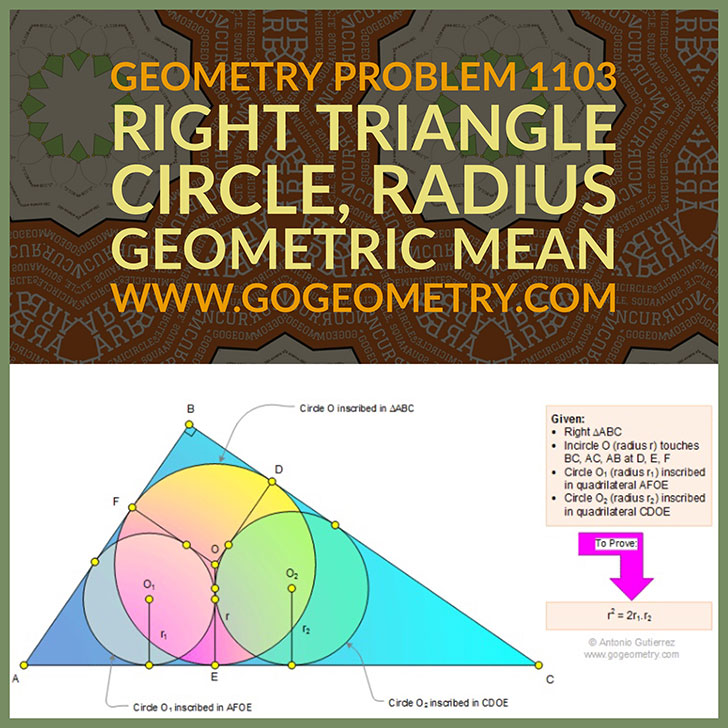Typography of Geometry Problem 1103: Right Triangle, Incircle, Inscribed Circle, Radius, Geometric Mean, iPad Apps

Proposition

In a right triangle ABC (see the figure below) incircle O (radius r) is tangent to BC, AC, and AB at D, E, and F, respectively. Circle O1 (radius r1) is inscribed in quadrilateral AFOE and circle O2 (radius r2) is inscribed in quadrilateral CDOE. Prove that r2 = 2r1.r2.